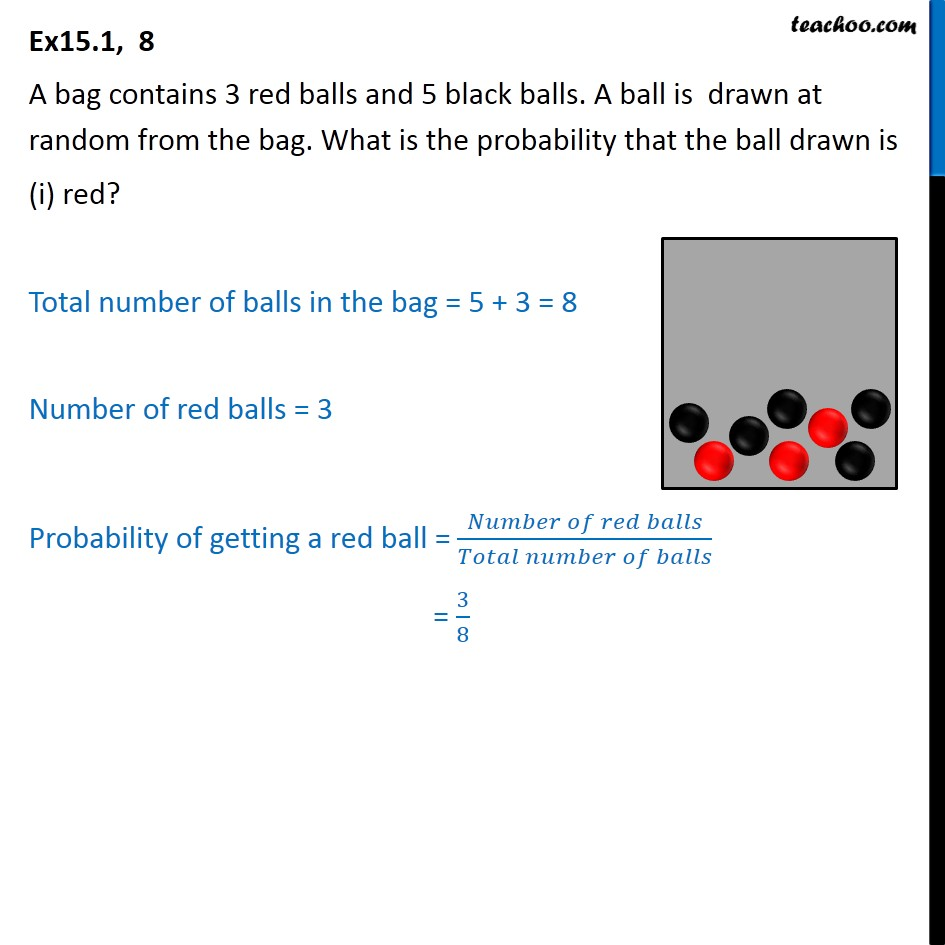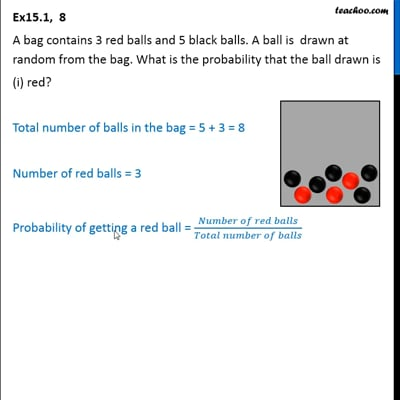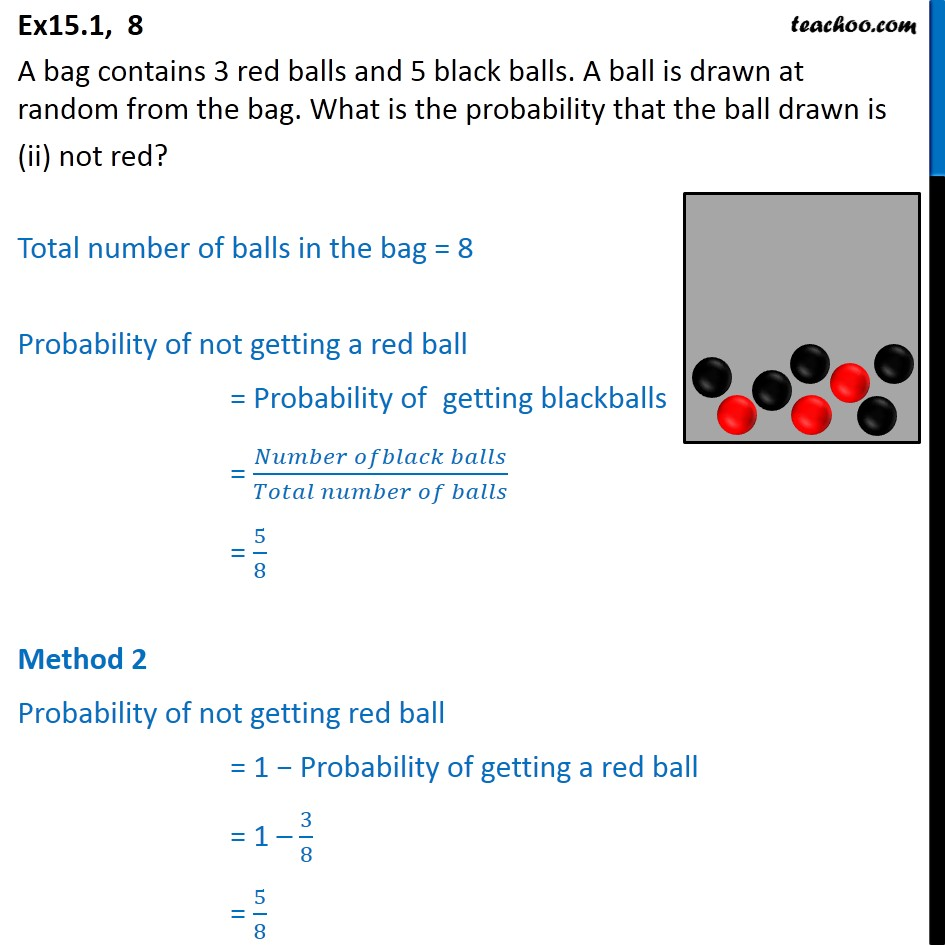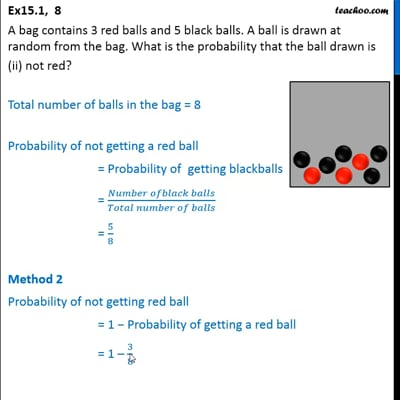Ex 15.1

Chapter 15 Class 10 Probability
Serial order wiseThis video is only available for Teachoo black usersThis video is only available for Teachoo black users

Solve all your doubts with Teachoo Black (new monthly pack available now!)

### Transcript

Ex15.1, 8 A bag contains 3 red balls and 5 black balls. A ball is drawn at random from the bag. What is the probability that the ball drawn is (i) red? Total number of balls in the bag = 5 + 3 = 8 Number of red balls = 3 Probability of getting a red ball = (𝑁𝑢𝑚𝑏𝑒𝑟 𝑜𝑓 𝑟𝑒𝑑 𝑏𝑎𝑙𝑙𝑠)/(𝑇𝑜𝑡𝑎𝑙 𝑛𝑢𝑚𝑏𝑒𝑟 𝑜𝑓 𝑏𝑎𝑙𝑙𝑠) = 3/8 Ex15.1, 8 A bag contains 3 red balls and 5 black balls. A ball is drawn at random from the bag. What is the probability that the ball drawn is (ii) not red? Total number of balls in the bag = 8 Probability of not getting a red ball = Probability of getting blackballs = (𝑁𝑢𝑚𝑏𝑒𝑟 𝑜𝑓𝑏𝑙𝑎𝑐𝑘 𝑏𝑎𝑙𝑙𝑠)/(𝑇𝑜𝑡𝑎𝑙 𝑛𝑢𝑚𝑏𝑒𝑟 𝑜𝑓 𝑏𝑎𝑙𝑙𝑠) = 5/8 Method 2 Probability of not getting red ball = 1 − Probability of getting a red ball = 1 – 3/8 = 5/8